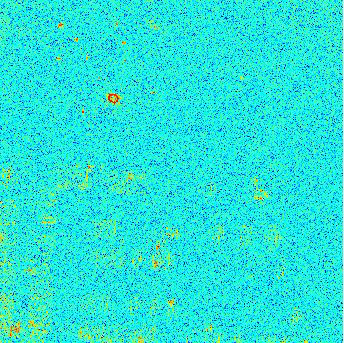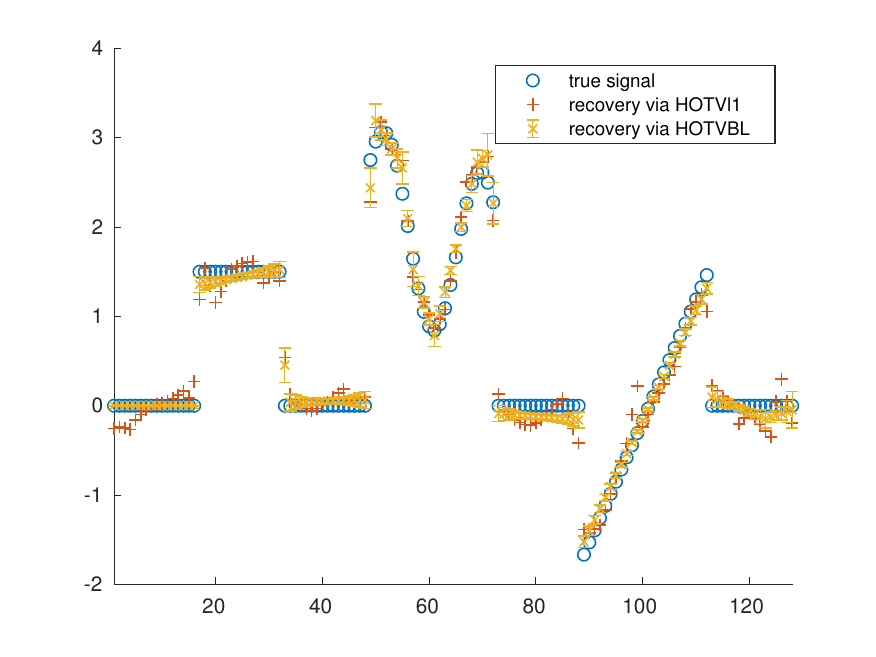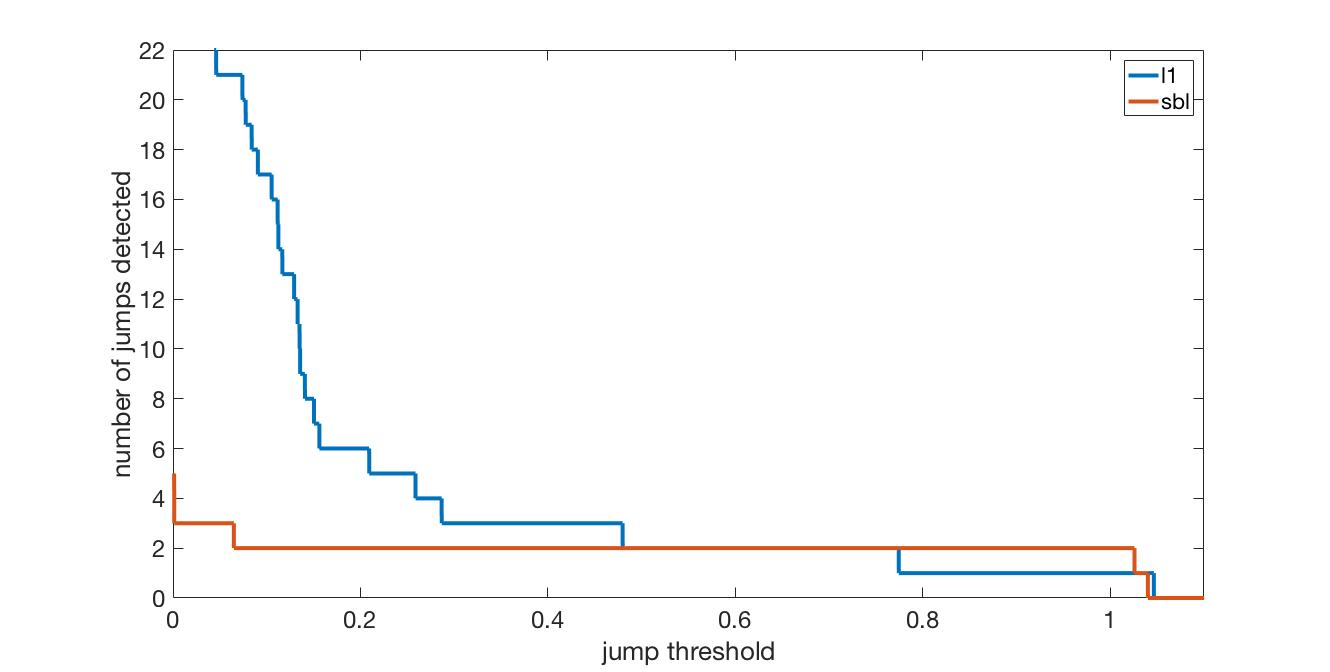RESEARCH
MCMC-based SAR Image Reconstruction

My most recent work has focused on quantifying uncertainty in synthetic aperture radar imaging. Typically in SAR imaging, only a single maximum a posteriori image estimate is retrieved. The maximum is not a categorically strong representative of the posterior, and provides no uncertainty quantification. Instead, we interrogate the posterior by sampling it using a Gibbs sampler. From the samples, confidence intervals are derived for each pixel value. To visualize, we display uniformly random draws from the confidence interval quickly in a gif. Below is a parking lot scene from the GOTCHA SAR data set from Air Force Research Laboratory. We are more confident in persistent features than those that flicker.High order total variation Bayesian learning, [pdf]

In this project, we modify the total variation operator in order to enable a Bayesian learning algorithm for piecewise smooth function recovery. The paper is submitted to Journal of Scientific Computing.Edge detection using sparse Bayesian learning, [pdf]

In this project we examine edge detection from non-uniform Fourier data. Below is a comparison of l1 regularization and SBL based on number of jumps detected in a particular problem for a given threshold on the jump height. This plot is derived from the reconstruction of the edge function from logarithmic Fourier sampling with zero-mean Gaussian noise with 0.02 standard deviation. The true number of jumps is two, and we see that SBL correctly identifies this for a wider range of thresholds.Binary weighted regularization for edge sparsity, [pdf]